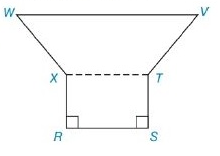Chapter 8.2, Problem 28E### Elementary Geometry for College St...

6th Edition
Daniel C. Alexander + 1 other
ISBN: 9781285195698

#### Solutions

Chapter
Section### Elementary Geometry for College St...

6th Edition
Daniel C. Alexander + 1 other
ISBN: 9781285195698
Textbook Problem
373 views

# In Exercises 27 to 30, find the area of the figure shown. Given: Hexagon RSTVWXwith W V - ∥ X T - ∥ R S - R S = 10 S T = 8 T V = 5 W V = 16 W X - ≅ V T - Find: A R S T V W XTo determine

To Find:

Area of the hexagon provided.

Explanation

Formula Used:

Split the hexagon into rectangle and trapezium and find the corresponding areas and sum it up.

Pythagorean theorem for the right angle triangle ABC for the hypotenuse AC,

AC2=AB2+BC2.

Area of rectangle =l×b

Area of trapezium =12h(b1+b2)

Calculation:

Let the vertices of the provided hexagon be R, S,T, V, W and X.

Let’s divide the quadrilateral into a rectangle and a trapezium. Draw a line from the point X to T.

Here RSTX forms a rectangle, the remaining XTVW forms the trapezium.

It is given that RS=10, ST=8, TV=5, WV=16 and WX-VT-.

Consider the rectangle TX,

Area of the rectangle RSTX is l×b=RS×ST=10×8=80 sq. units.

Consider the trapezium XTVW,

It is given that WX-VT-. The given trapezium is an isosceles trapezium.

Since RSTX is a rectangle, RS=TX=10

### Still sussing out bartleby?

Check out a sample textbook solution.

See a sample solution

#### The Solution to Your Study Problems

Bartleby provides explanations to thousands of textbook problems written by our experts, many with advanced degrees!

Get Started

#### Find more solutions based on key concepts